JMSLTM Numerical Library 7.2.0
com.imsl.math

• All Implemented Interfaces:
Serializable, Cloneable

```public class RadialBasis
extends Object
implements Serializable, Cloneable```

RadialBasis computes a least-squares fit to scattered data in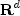, where d is the dimension. More precisely, we are given data points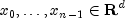and function values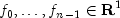The radial basis fit to the data is a function F which approximates the above data in the sense that it minimizes the sum-of-squares error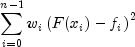where w are the weights. Of course, we must restrict the functional form of F. Here we assume it is a linear combination of radial functions: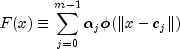The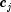are the centers.

A radial function,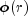, maps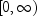into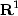. The default radial function is the Hardy multiquadric,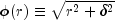with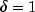. An alternate radial function is the Gaussian,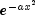.

By default, the centers are points in a Faure sequence, scaled to cover the box containing the data.

Two `update` methods allow the user to specify weights for each data point in the approximation scheme. In this way the user can influence the fit of the radial basis function. For example, if weights are in the range [0,1] then 0-weighted points are effectively removed from computations and 1-weighted points will have more influence than any others. When the number of centers equals the number of data points, the RBF fit will be "exact", otherwise it will be an approximation (useful for large or noisy data sets). Provided the ratios of the weights are not too extreme, weights will not appreciably change the accuracy of the fit to the data, but they will affect the shape of the approximating function away from the data: Greater weights result in greater influence at greater distances.

Example, Serialized Form
• ### Nested Class Summary

Nested Classes
Modifier and Type Class and Description
`static interface ` `RadialBasis.Function`
Public interface for the user supplied function to the ```RadialBasis ``` object.
`static class ` `RadialBasis.Gaussian`
The Gaussian basis function,.
`static class ` `RadialBasis.HardyMultiquadric`
The Hardy multiquadric basis function,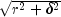.
• ### Constructor Summary

Constructors
Constructor and Description
```RadialBasis(int nDim, int nCenters)```
Creates a new instance of RadialBasis.
• ### Method Summary

Methods
Modifier and Type Method and Description
`ANOVA` `getANOVA()`
Returns the ANOVA statistics from the linear regression.
`RadialBasis.Function` `getRadialFunction()`
`double[]` `gradient(double[] x)`
`void` `setRadialFunction(RadialBasis.Function radialFunction)`
`void` ```update(double[][] x, double[] f)```
Adds a set of data points, all with weight = 1.
`void` ```update(double[][] x, double[] f, double[] w)```
Adds a set of data points with user-specified weights.
`void` ```update(double[] x, double f)```
Adds a data point with weight = 1.
`void` ```update(double[] x, double f, double w)```
Adds a data point with a specified weight.
`double` `value(double[] x)`
Returns the value of the radial basis approximation at a point.
`double[]` `value(double[][] x)`
Returns the value of the radial basis at a point.
• ### Methods inherited from class java.lang.Object

`clone, equals, finalize, getClass, hashCode, notify, notifyAll, toString, wait, wait, wait`
• ### Constructor Detail

```public RadialBasis(int nDim,
int nCenters)```
Creates a new instance of RadialBasis.
Parameters:
`nDim` - an `int` specifying the number of dimensions.
`nCenters` - an `int` specifying the number of centers.
• ### Method Detail

• #### getANOVA

`public ANOVA getANOVA()`
Returns the ANOVA statistics from the linear regression.
Returns:
an `ANOVA` table and related statistics
`LinearRegression`, `ANOVA`

`public RadialBasis.Function getRadialFunction()`
Returns:
a `RadialBasis.Function` which is the current radial function.

`public double[] gradient(double[] x)`
Parameters:
`x` - is a `double` array containing the locations of the data point at which the approximation's gradient is to be computed.
Returns:
a `double` array, of length `nDim` containing the value of the gradient of the radial basis approximation at x.

`public void setRadialFunction(RadialBasis.Function radialFunction)`
Parameters:
`radialFunction` - a `RadialBasis.Function` to be used in the approximation. The default is Hardy Multiquadric with.
• #### update

```public void update(double[][] x,
double[] f)```
Adds a set of data points, all with weight = 1.
Parameters:
`x` - is a `double` matrix of size n by nDim containing the locations of the data points for each dimension.
`f` - is a `double` array containing the function values at the data points.
• #### update

```public void update(double[][] x,
double[] f,
double[] w)```
Adds a set of data points with user-specified weights.
Parameters:
`x` - is a `double` matrix of size n by nDim containing the locations of the data points for each dimension.
`f` - is a `double` array containing the function values at the data points.
`w` - is a `double` array containing the weights associated with the data points.
• #### update

```public void update(double[] x,
double f)```
Adds a data point with weight = 1.
Parameters:
`x` - is a `double` array containing the locations of the data point.
`f` - is a `double` containing the function value at the data point.
• #### update

```public void update(double[] x,
double f,
double w)```
Adds a data point with a specified weight.
Parameters:
`x` - is a `double` array containing the locations of the data point.
`f` - is a `double` containing the function value at the data point.
`w` - is a `double` containing the weight of this data point.
• #### value

`public double value(double[] x)`
Returns the value of the radial basis approximation at a point.
Parameters:
`x` - is a `double` array containing the locations of the data point at which the approximation is to be computed.
Returns:
a `double` containing the value of the radial basis approximation at x.
• #### value

`public double[] value(double[][] x)`
Returns the value of the radial basis at a point.
Parameters:
`x` - a `double` matrix of size n by nDim containing the points at which the radial basis is to be evaluated.
Returns:
a `double` array giving the value of the radial basis at the point `x`
JMSLTM Numerical Library 7.2.0